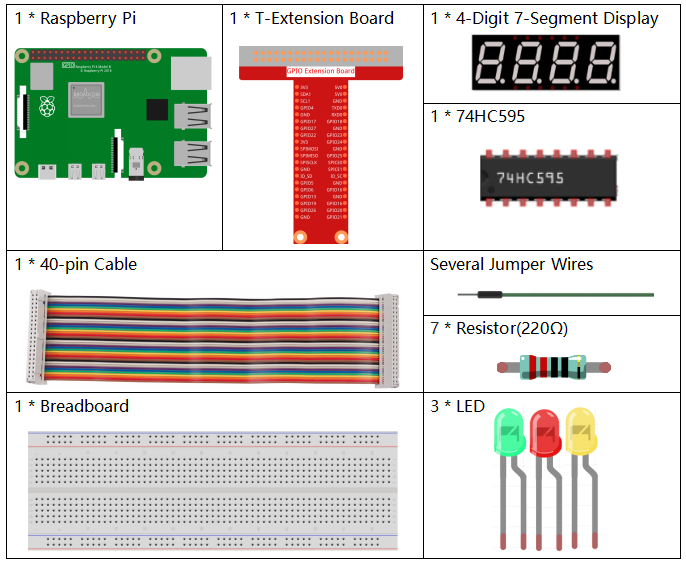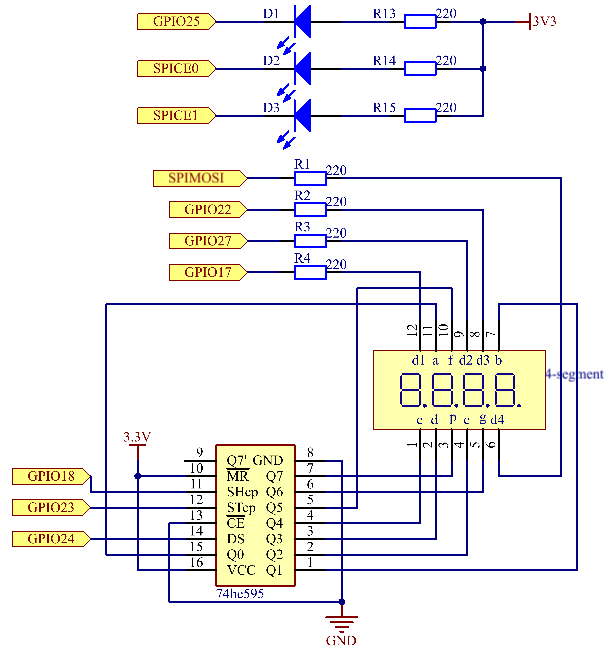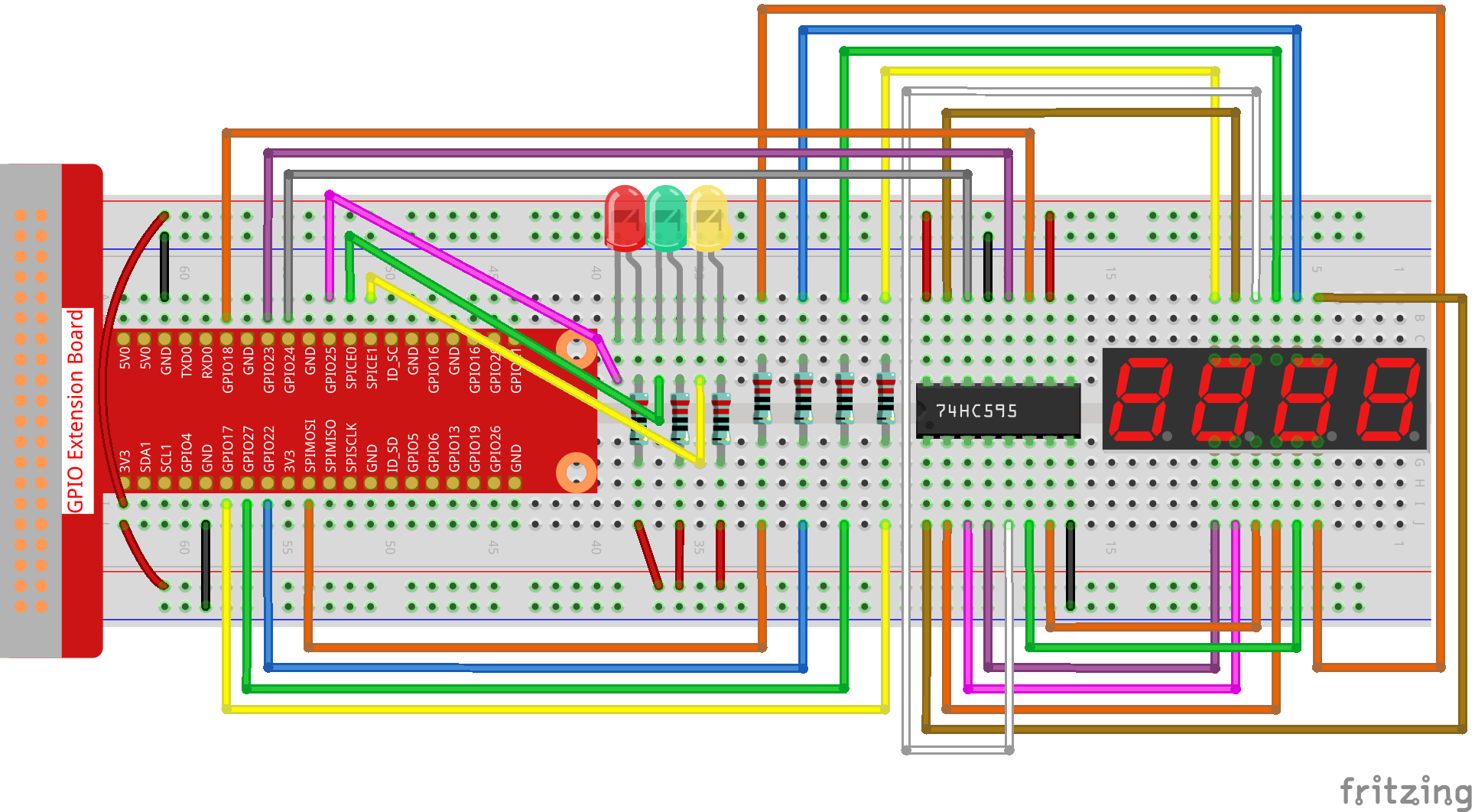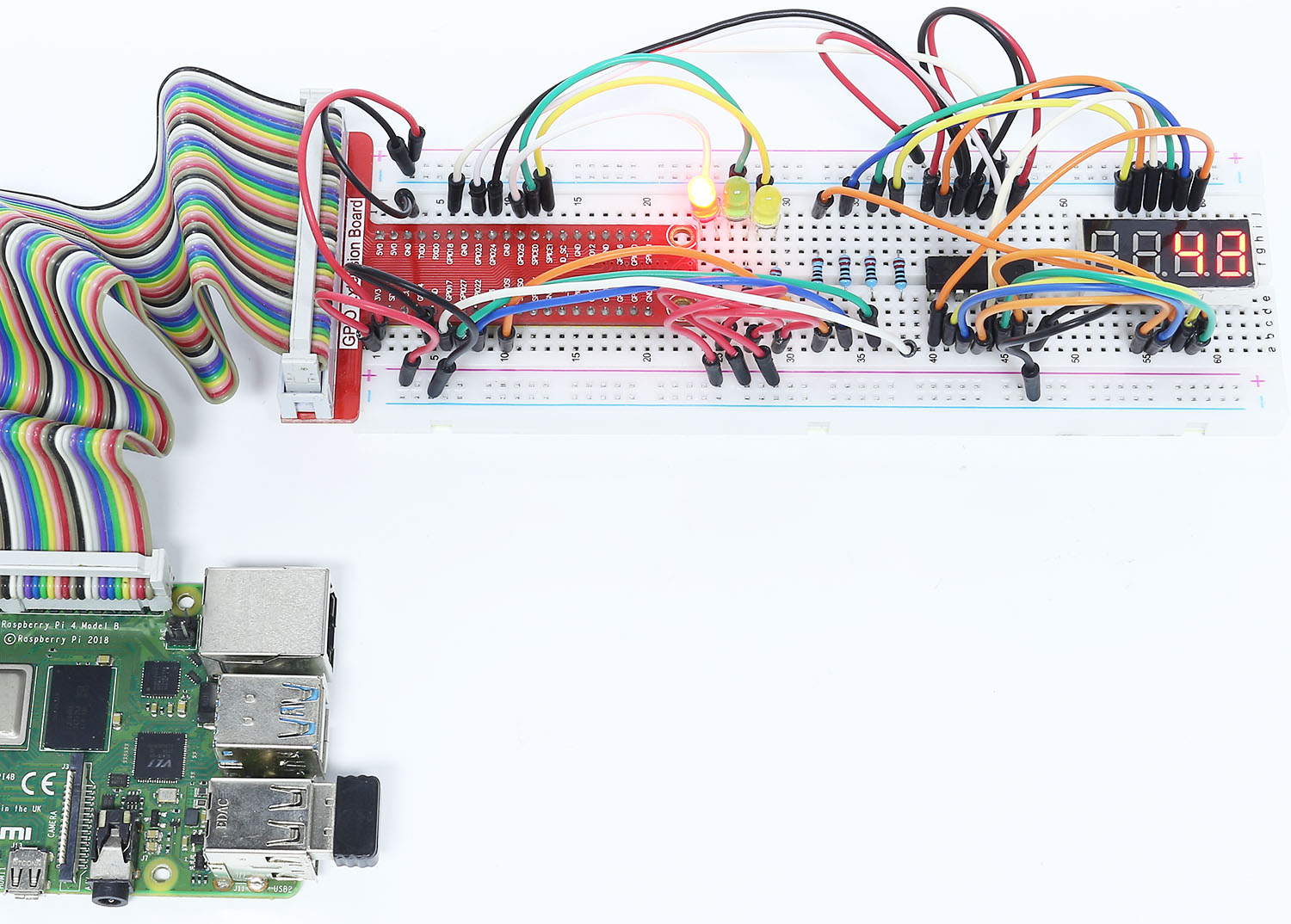# 3.1.7 Traffic Light¶

## Introduction¶

In this project, we will use LED lights of three colors to realize the change of traffic lights and a four-digit 7-segment display will be used to display the timing of each traffic state.

## Components¶## Schematic Diagram¶

 T-Board Name physical wiringPi BCM GPIO17 Pin 11 0 17 GPIO27 Pin 13 2 27 GPIO22 Pin 15 3 22 SPIMOSI Pin 19 12 10 GPIO18 Pin 12 1 18 GPIO23 Pin 16 4 23 GPIO24 Pin 18 5 24 GPIO25 Pin 22 6 25 SPICE0 Pin 24 10 8 SPICE1 Pin 26 11 7## Experimental Procedures¶

Step 1: Build the circuit.### For C Language Users¶

Step 2: Change directory.

```cd ~/davinci-kit-for-raspberry-pi/c/3.1.7/
```

Step 3: Compile.

```gcc 3.1.7_TrafficLight.c -lwiringPi
```

Step 4: Run.

```sudo ./a.out
```

As the code runs, LEDs will simulate the color changing of traffic lights. Firstly, the red LED lights up for 60s, then the green LED lights up for 30s; next, the yellow LED lights up for 5s. After that, the red LED lights up for 60s once again. In this way, this series of actions will be executed repeatedly.

Note

If it does not work after running, or there is an error prompt: "wiringPi.h: No such file or directory", please refer to C code is not working?.

Code Explanation

```#define     SDI     5
#define     RCLK    4
#define     SRCLK    1

const int placePin[] = {12, 3, 2, 0};
unsigned char number[] = {0xc0, 0xf9, 0xa4, 0xb0, 0x99, 0x92, 0x82, 0xf8, 0x80, 0x90};

void pickDigit(int digit);
void hc595_shift(int8_t data);
void clearDisplay();
void display();
```

These codes are used to realize the function of number display of 4-Digit 7-Segment Displays. Refer to 1.1.5 4-Digit 7-Segment Display of the document for more details. Here, we use the codes to display countdown of traffic light time.

```const int ledPin[]={6,10,11};

int colorState = 0;

void lightup()
{
for(int i=0;i<3;i++){
digitalWrite(ledPin[i],HIGH);
}
digitalWrite(ledPin[colorState],LOW);
}
```

The codes are used to switch the LED on and off.

```int greenLight = 30;
int yellowLight = 5;
int redLight = 60;
int colorState = 0;
char *lightColor[]={"Red","Green","Yellow"};
int counter = 60;

void timer(int  timer1){       //Timer function
if(timer1 == SIGALRM){
counter --;
alarm(1);
if(counter == 0){
if(colorState == 0) counter = greenLight;
if(colorState == 1) counter = yellowLight;
if(colorState == 2) counter = redLight;
colorState = (colorState+1)%3;
}
printf("counter : %d \t light color: %s \n",counter,lightColor[colorState]);
}
}
```

The codes are used to switch the timer on and off. Refer to 1.1.5 4-Digit 7-Segment Display for more details. Here, when the timer returns to zero, colorState will be switched so as to switch LED, and the timer will be assigned to a new value.

```void loop()
{
while(1){
display();
lightup();
}
}

int main(void)
{
//…
signal(SIGALRM,timer);
alarm(1);
loop();
return 0;
}
```

The timer is started in the main() function. In loop() function, use while(1) loop and call the functions of 4-Digit 7-Segment and LED.

### For Python Language Users¶

Step 2: Change directory.

```cd ~/davinci-kit-for-raspberry-pi/python/
```

Step 3: Run.

```sudo python3 3.1.7_TrafficLight.py
```

As the code runs, LEDs will simulate the color changing of traffic lights. Firstly, the red LED lights up for 60s, then the green LED lights up for 30s; next, the yellow LED lights up for 5s. After that, the red LED lights up for 60s once again. In this way, this series of actions will be executed repeatedly. Meanwhile, the 4-digit 7-segment display displays the countdown time continuously.

Code

Note

You can Modify/Reset/Copy/Run/Stop the code below. But before that, you need to go to source code path like `davinci-kit-for-raspberry-pi/python`.

```import RPi.GPIO as GPIO
import time

#define the pins connect to 74HC595
SDI   = 24      #serial data input(DS)
RCLK  = 23     #memory clock input(STCP)
SRCLK = 18      #shift register clock input(SHCP)
number = (0xc0,0xf9,0xa4,0xb0,0x99,0x92,0x82,0xf8,0x80,0x90)

placePin = (10,22,27,17)
ledPin =(25,8,7)

greenLight = 30
yellowLight = 5
redLight = 60
lightColor=("Red","Green","Yellow")

colorState=0
counter = 60
timer1 = 0

def setup():
GPIO.setmode(GPIO.BCM)
GPIO.setup(SDI, GPIO.OUT)
GPIO.setup(RCLK, GPIO.OUT)
GPIO.setup(SRCLK, GPIO.OUT)
for pin in placePin:
GPIO.setup(pin,GPIO.OUT)
for pin in ledPin:
GPIO.setup(pin,GPIO.OUT)
global timer1
timer1.start()

def clearDisplay():
for i in range(8):
GPIO.output(SDI, 1)
GPIO.output(SRCLK, GPIO.HIGH)
GPIO.output(SRCLK, GPIO.LOW)
GPIO.output(RCLK, GPIO.HIGH)
GPIO.output(RCLK, GPIO.LOW)

def hc595_shift(data):
for i in range(8):
GPIO.output(SDI, 0x80 & (data << i))
GPIO.output(SRCLK, GPIO.HIGH)
GPIO.output(SRCLK, GPIO.LOW)
GPIO.output(RCLK, GPIO.HIGH)
GPIO.output(RCLK, GPIO.LOW)

def pickDigit(digit):
for i in placePin:
GPIO.output(i,GPIO.LOW)
GPIO.output(placePin[digit], GPIO.HIGH)

def timer():        #timer function
global counter
global colorState
global timer1
timer1.start()
counter-=1
if (counter is 0):
if(colorState is 0):
counter= greenLight
if(colorState is 1):
counter=yellowLight
if (colorState is 2):
counter=redLight
colorState=(colorState+1)%3
print ("counter : %d    color: %s "%(counter,lightColor[colorState]))

def lightup():
global colorState
for i in range(0,3):
GPIO.output(ledPin[i], GPIO.HIGH)
GPIO.output(ledPin[colorState], GPIO.LOW)

def display():
global counter

a = counter % 10000//1000 + counter % 1000//100
b = counter % 10000//1000 + counter % 1000//100 + counter % 100//10
c = counter % 10000//1000 + counter % 1000//100 + counter % 100//10 + counter % 10

if (counter % 10000//1000 == 0):
clearDisplay()
else:
clearDisplay()
pickDigit(3)
hc595_shift(number[counter % 10000//1000])

if (a == 0):
clearDisplay()
else:
clearDisplay()
pickDigit(2)
hc595_shift(number[counter % 1000//100])

if (b == 0):
clearDisplay()
else:
clearDisplay()
pickDigit(1)
hc595_shift(number[counter % 100//10])

if(c == 0):
clearDisplay()
else:
clearDisplay()
pickDigit(0)
hc595_shift(number[counter % 10])

def loop():
while True:
display()
lightup()

def destroy():   # When "Ctrl+C" is pressed, the function is executed.
global timer1
GPIO.cleanup()
timer1.cancel()      #cancel the timer

if __name__ == '__main__': # Program starting from here
setup()
try:
loop()
except KeyboardInterrupt:
destroy()
```

Code Explanation

```SDI   = 24      #serial data input(DS)
RCLK  = 23     #memory clock input(STCP)
SRCLK = 18      #shift register clock input(SHCP)
number = (0xc0,0xf9,0xa4,0xb0,0x99,0x92,0x82,0xf8,0x80,0x90)
placePin = (10,22,27,17)

def clearDisplay():
def hc595_shift(data):
def pickDigit(digit):
def display():
```

These codes are used to realize the function of number display of 4-Digit 7-Segment. Refer to 1.1.5 4-Digit 7-Segment Display of the document for more details. Here, we use the codes to display countdown of traffic light time.

```ledPin =(25,8,7)
colorState=0

def lightup():
global colorState
for i in range(0,3):
GPIO.output(ledPin[i], GPIO.HIGH)
GPIO.output(ledPin[colorState], GPIO.LOW)
```

The codes are used to switch the LED on and off.

```greenLight = 30
yellowLight = 5
redLight = 60
lightColor=("Red","Green","Yellow")

colorState=0
counter = 60
timer1 = 0

def timer():        #timer function
global counter
global colorState
global timer1
timer1.start()
counter-=1
if (counter is 0):
if(colorState is 0):
counter= greenLight
if(colorState is 1):
counter=yellowLight
if (colorState is 2):
counter=redLight
colorState=(colorState+1)%3
print ("counter : %d    color: %s "%(counter,lightColor[colorState]))
```

The codes are used to switch the timer on and off. Refer to 1.1.5 4-Digit 7-Segment Display for more details. Here, when the timer returns to zero, colorState will be switched so as to switch LED, and the timer will be assigned to a new value.

```def setup():
# ...
global timer1
timer1.start()

def loop():
while True:
display()
lightup()

def destroy():   # When "Ctrl+C" is pressed, the function is executed.
global timer1
GPIO.cleanup()
timer1.cancel()      #cancel the timer

if __name__ == '__main__': # Program starting from here
setup()
try:
loop()
except KeyboardInterrupt:
destroy()
```

In setup() function, start the timer. In loop() function, a ```while True``` is used: call the relative functions of 4-Digit 7-Segment and LED circularly.

## Phenomenon Picture¶